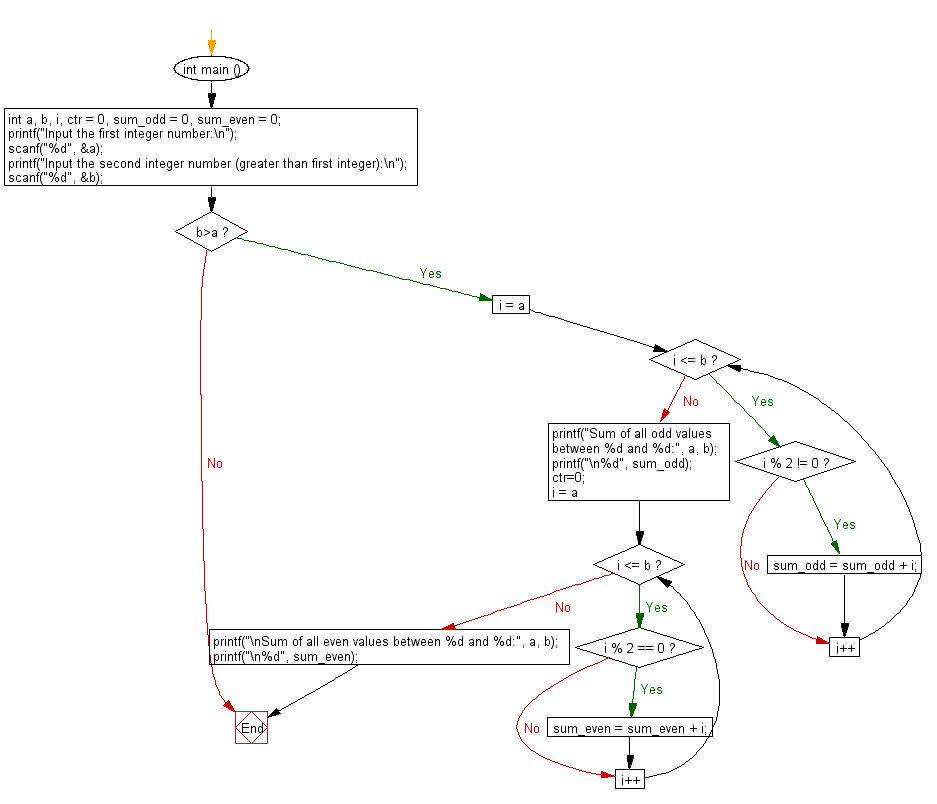﻿ C Program: Sum of all odd, even values between two integers - w3resource

# C Exercises: Sum of all odd, even values between two integers

## C Basic Declarations and Expressions: Exercise-108 with Solution

Write a C program that reads two integer values and calculates the sum of all odd numbers between them.

Sample Solution:

C Code:

``````#include <stdio.h>
int main () {
// Declare variables for input, counters, and sum of odd and even numbers
int a, b, i, ctr = 0, sum_odd = 0, sum_even = 0;

// Prompt the user to input the first integer number
printf("Input the first integer number:\n");
scanf("%d", &a);

// Prompt the user to input the second integer number (greater than the first integer)
printf("Input the second integer number (greater than first integer):\n");
scanf("%d", &b);

// Check if b is greater than a
if (b > a)
{
// Calculate the sum of all odd values between a and b
for (i = a; i <= b; i++){

if (i % 2 != 0){
sum_odd = sum_odd + i;
}
}

// Print the sum of all odd values
printf("Sum of all odd values between %d and %d:", a, b);
printf("\n%d", sum_odd);

// Reset counter
ctr = 0;

// Calculate the sum of all even values between a and b
for (i = a; i <= b; i++){
if (i % 2 == 0){
sum_even = sum_even + i;
}
}

// Print the sum of all even values
printf("\nSum of all even values between %d and %d:", a, b);
printf("\n%d", sum_even);
}
}
``````

Sample Output:

```Input the first integer number:
25
Input the second integer number (greater than first integer):
45
Sum of all odd values between 25 and 45:
385
Sum of all even values between 25 and 45:
350
```

Flowchart:C programming Code Editor:

What is the difficulty level of this exercise?

Test your Programming skills with w3resource's quiz.

﻿